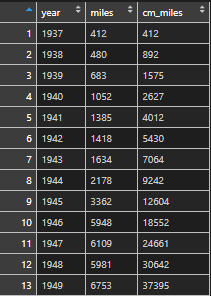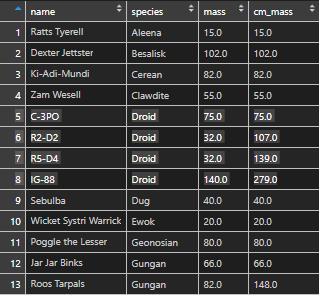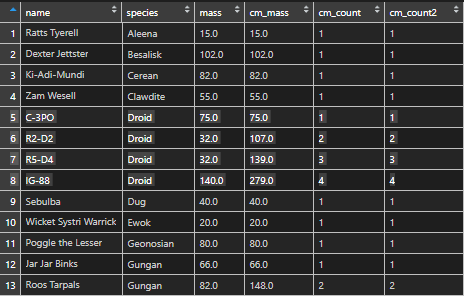# Cumulative sum or count in R

Here is how to calculate cumulative sum or count by using R built-in datasets.

## Cumulative sum in R

Here is data from the R built-in airpassanger dataset. This data comes in time-series format and first of all, I will create a data frame.

The cumulative sum is calculated by using function cumsum.

```am <- data.frame("year" = time(airmiles), "miles" = airmiles)

am\$cm_miles <- cumsum(am\$miles)```## Cummulative calculation within group in R

Sometimes cumulative sum is needed within the group. For example withing year, month or whatever.

You can do it by adding group_by from dplyr.

```require(dplyr)

# select data from Starwars dataset
sw <- starwars %>%
select(name, species, mass) %>%
filter(!is.na(mass)) %>%
arrange(species)

# calculate cumulative sum of mass by species
sw <- sw %>%
group_by(species) %>%
mutate("cm_mass" = cumsum(mass))```In this example, I was actually running into dplyr unused argument error, because select is also in MASS. Check out this post on how to deal with that.

## Cummulative count or group index in R

You can do it in at least two different ways. I’m continuing the previous example.

```# create column with 1
sw\$rec <- 1

# calculate cumulative count
sw <- sw %>%
group_by(species) %>%
mutate("cm_count" = cumsum(rec)) %>%
select(-rec) # remove column that is not needed

library(data.table)

sw\$cm_count2 <- rowid(sw\$species)```Posted

in